HOME / Applications / A Spoke Resonator for Linear Accelerators

# A Spoke Resonator for Linear Accelerators

Used Tools:## Spoke Resonator

A resonator is a device that oscillates naturally at certain frequencies (also called as natural frequencies or resonant frequencies) with greater amplitudes than at other frequencies. The oscillations can be either acoustic, mechanical or electromagnetic in nature. In this application note, we will assume the oscillations to be electromagnetic in nature. These types of resonators are called Cavity Resonators. It is usually a hollow metallic structure that confines electromagnetic fields within it. The EM waves bounce back and forth between the walls of the cavity and at the cavity’s resonant frequencies they reinforce to form standing waves. They are widely used in oscillators and transmitters to create microwave signals and as filters in radar equipment, microwave relay station etc.  RF cavities can also manipulate charged particles passing through them by application of acceleration voltage and are thus used in particle accelerators and magnetrons. A spoke resonator as shown in Figure 1 is a special type of a cavity resonator that is used in particle acceleration.

## SolidWorks Model

Figure 1 shows a spoke resonator created using SolidWorks.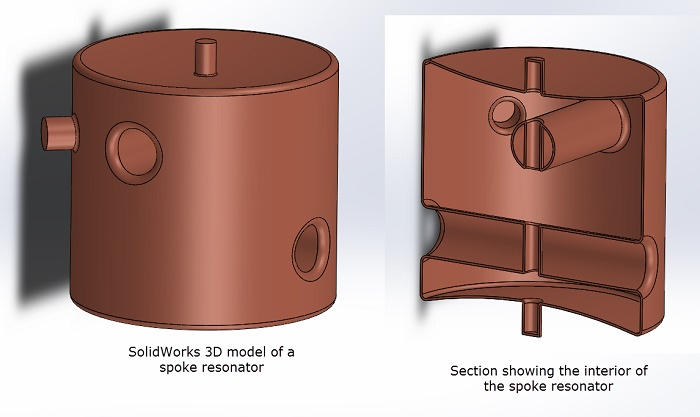Figure 1 - Spoke resonator designed in SolidWorks

This resonator is designed to have a first resonant frequency of 325 MHz. The objectives of the EM simulation are two folds –

1. To compute the first resonant frequency
2. To compute the quality factor (Qo factor)

The simulation is carried out using HFWorks which is a full 3D, CAD embedded high frequency simulation package for SolidWorks.

## Resonant Frequency Computation

The simulation study used to compute resonant frequencies is called a Resonance study, Figure 2. HFWorks can compute several resonant frequencies for any structure. For the spoke resonator, we compute the first mode (or the first resonant frequency) and compare it against the test results. HFWorks calculates the first resonant frequency, Figure 3 to be 323.02 MHz. This compares very well with the test result of 324.73 MHz.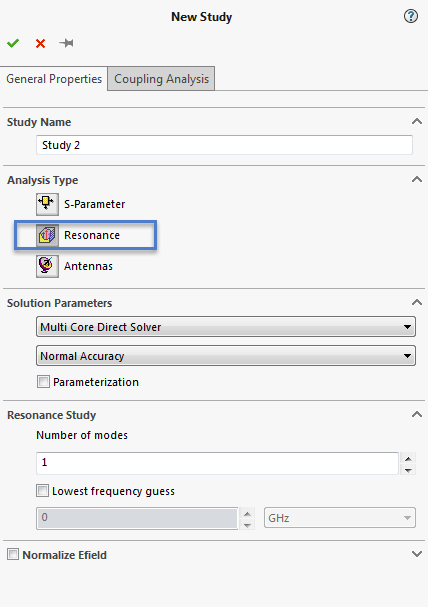Figure 2- Creating a Resonance study in HFWorks

Table 1 - Comparing first resonance frequency

HFWorks result Test result
First resonant frequency (MHz) 323.02 324.73

## Quality Factor (Qo) Computation

In addition to the resonant frequencies, HFWorks computes the quality factor (Qo) and the Stored Energy for the resonator for each of the resonant frequencies. Figure 4 shows the table generated by HFWorks for the first resonant frequency.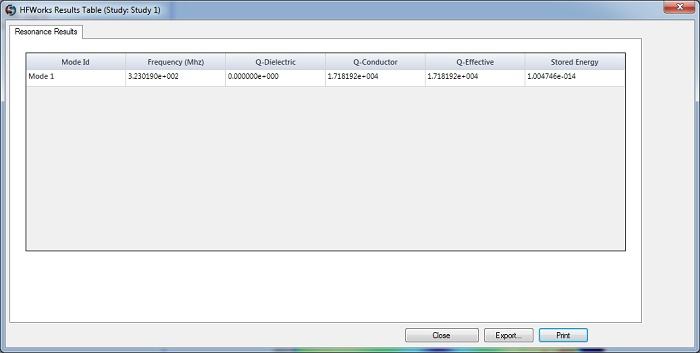Figure 3 - Table showing 1st resonant frequency and Qo

## 3D Fields

Figure 5 shows the distribution of the Electric Field inside the resonator as a section plot. Figure 6 shows the Magnetic Field Intensity inside the resonator as a section plot. The 3D field results help the engineer to qualitatively analyze the behaviour of the resonator.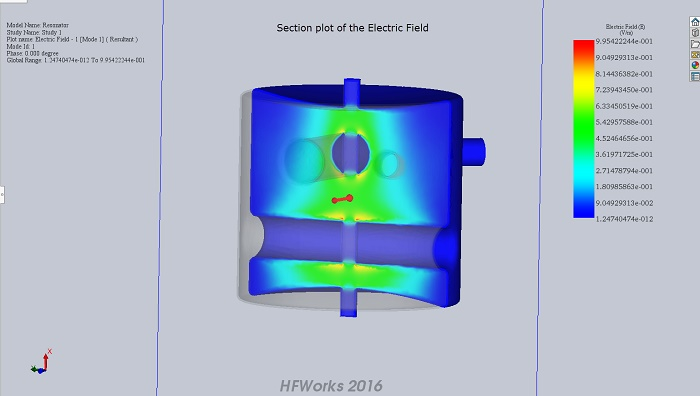Figure 4 - Section plot of Electric Field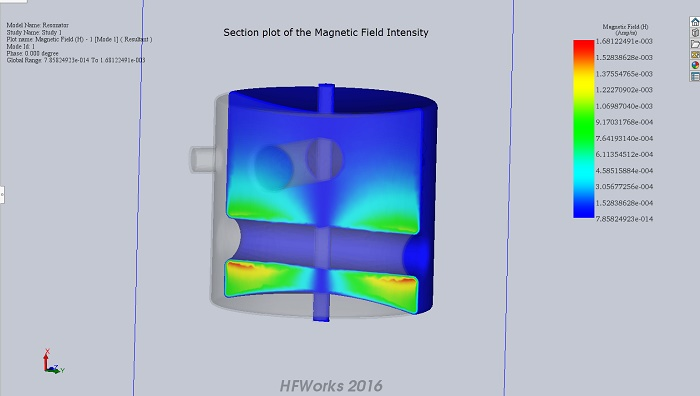Figure 5 - Section plot of Magnetic Field Intensity

## Conclusion

The resonant frequency computed by HFWorks is within 0.5 % of the test value. The Q factor (Qo) for the resonator was computed to be 1.72e4. HFWorks can compute the resonant frequencies of any structure and can be used to design resonators for various RF and microwave applications including particle acceleration.

## References

G. Lanfranco , G. Apollinari, I.V. Gonin, T.N. Khabiboulline, G. Romanov, R. Wagner (2007). PRODUCTION OF 325 MHZ SINGLE SPOKE RESONATORS AT FNAL. Proceedings of PAC07, Albuquerque, New Mexico, USA. Retrieved from https://accelconf.web.cern.ch/accelconf/p07/PAPERS/WEPMN099.PDF